# GSEB Solutions Class 9 Maths Chapter 7 Triangles Ex 7.5

Gujarat Board GSEB Solutions Class 9 Maths Chapter 7 Triangles Ex 7.5 Textbook Questions and Answers.

## Gujarat Board Textbook Solutions Class 9 Maths Chapter 7 Triangles Ex 7.5

Question 1.
ABC is a triangle. Locate a point in the interior of ΔABC which is equidistant from all the vertices of ΔABC.
Solution:
Draw perpendicular bisector of any two sides of ΔABC. The point of intersection of these perpendicular bisectors is equidistant from all the vertices of Hence point O will be the centre of the circle which passes through all vertices.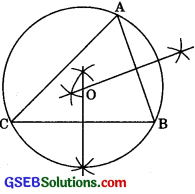Question 2.
In a triangle locate a point in its interior which is equidistant from all the sides of the triangle.
Solution:
The draw angle bisector of any two angles of a triangle. Where the point of intersection of these angle bisectors would be equidistant from all the three sides of the triangle. Thus, point O is the in centre of the triangle.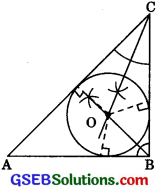Question 3.
In a huge park, people are concentrated at three points (See figure)
A: where there are different slides and swings for children.B: near which a man-made lake is situated.
C: which is near to a large parking and exit. Where should an ice-cream parlour be set up so that the maximum number of persons can approach it?
(Hint: the parlour should be equidistant from A, B and C)
Solution:
If ice-cream parlour is situated on the circumcentre of the circle, then the maximum number of people will reach. It will be equidistant from each vertex A, B and C.Question 4.
Complete the hexagonal and star shaped Rangolies (See figure (i) and (ii)) by filling them with as many equilateral triangles of side 1 cm as you can. Count the number of triangles in each case. Which has more triangles?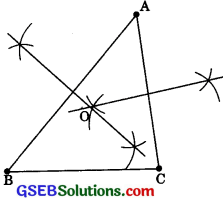Solution:
(i) Area of ΔOAB = $$\frac{\sqrt{3}}{4}$$ side2 =$$\frac{\sqrt{3}}{4}$$ x 52
$$\frac{\sqrt{3} \times 25}{4}$$
Area of hexagonal shaped Rangoli = $$\frac{6 \times 25 \sqrt{3}}{4}$$ = $$\frac{75}{2} \sqrt{3}$$
Area of an equilateral triangle of side 1 cm
= $$\frac{\sqrt{3}}{4}$$ x 12 = $$\frac{\sqrt{3}}{4}$$ cm2
No. of equilateral triangles of side 1 cm formed in hexagonal Rangoli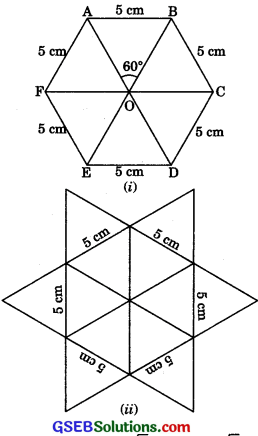(ii) Star shaped Rangoli has 12 equilateral triangles of side 5 cm each.
∴ Area of star shaped Rangoli = 12 x $$\frac{\sqrt{3}}{4}$$ x 52
= 12 x $$\frac{\sqrt{3}}{4}$$ x 25 = 75 $$\sqrt{3}$$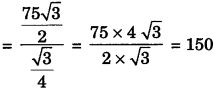No. of equilateral triangles of side 1 cm formed in stare shaped Rangoli= 75 x 4 = 300
Therefore, star shaped Rangoli has more triangles.